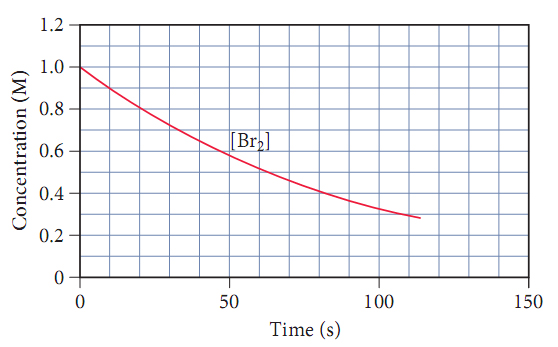# Problem: Consider the following reaction: H2(g) + Br2(g) → 2HBr. The graph below shows the concentration of Br2 as a function of time.Make a rough sketch of a curve representing the concentration of HBr as a function of time. Assume that the initial concentration of HBr is zero.

⚠️Our tutors found the solution shown to be helpful for the problem you're searching for. We don't have the exact solution yet.

###### Problem Details

Consider the following reaction: H2(g) + Br2(g) → 2HBr. The graph below shows the concentration of Br2 as a function of time.Make a rough sketch of a curve representing the concentration of HBr as a function of time. Assume that the initial concentration of HBr is zero.